Computer simulation with the help of computational methods, such as finite element methodfinite difference method, and finite volume method, enables prediction of the behavior of objects like buildings, gas wells, road embankments, pipeline supports, etc. in real use conditions. Simulation helps ensure the effectiveness and reliability of design solutions with minimal time and material costs.

Computer simulation of artificial ground freezing requires the use of specialized software Frost 3D, where the heat equation with boundary conditions is solved via numerical methods:Where:is temperature;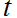is time;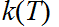is dependence of thermal conductivity on temperature;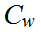is volumetric heat capacity of water;is filtration velocity;denotes sources and drains of heat;is heat transfer coefficient;is temperature of environment;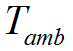is temperature of heat radiation source;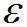is emissivity factor of ground surface;is Stefan–Boltzmann constant;is heat flow (e.g. from evaporator of a cooling device).

Apparent heat capacity and thermal conductivity of the ground can be specified in terms of temperature dependence or calculated by respective equations.Dependence of heat capacity on temperatureDependence of thermal conductivity on temperature

The following equations for the computation of effective heat capacity and thermal conductivity have become the most widely used: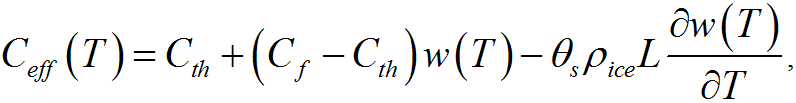Where: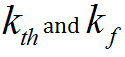are the values of thermal conductivity of thawed and frozen ground respectively;is volumetric moisture content of ground;is dependence of ice content on temperature that can be approximated by the following empirical dependence:Where: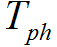is temperature of water-ice phase transition;is empirical coefficient that determines steepness of ice content change from temperature in the vicinity of phase transition.

The heat equation with boundary conditions is solved for a three-dimensional area, which is analyzed during the design process of a construction object. This area usually includes geological and lithological ground layers, designed basements and foundations, heat-insulating layers, cooling devices, and other elements.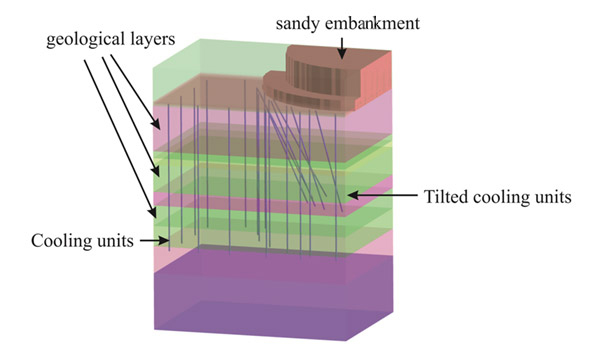Simulation area for ground freezing

As a rule, ground surface is taken as the upper boundary of the simulation area, whereas the lower boundary is set deep enough to minimize its influence on thermal processes in the area of interest to the design engineer.

The conditions of heat transfer with the surrounding environment, determined by air temperature and heat transfer coefficient, are specified on the upper boundary of the simulation area. In the presence of heat radiation sources such as gas flares, the temperature of the heat radiation source and the ground surface emissivity factor are additionally specified. The impact of snow cover on the heat transfer of ground surface is accounted for by specifying the variation in snow cover thickness and respective thermal conductivity over time.

Ground temperature is specified at the lower boundary of the simulation area in accordance with thermometric data. As a rule, the side surface of the simulation area is specified with zero heat flow. At the same time, the side boundaries of the simulation area should be located far enough away from the area of interest to the design engineer so that they did not affect the calculation of the thermal processes.Ground thermal regime prediction: setting boundary conditions

It should be noted that the simulation area consists of heterogeneous ground layers with different physical properties – heat capacity, thermal conductivity, density, and moisture content. In this case, it is necessary to construct a three-dimensional simulation area for the computer simulation of artificial ground freezing, which takes into account the actual distribution of ground layers with different physical properties. Such a distribution of ground layers should be built on the basis of data obtained from exploratory boreholes. In a complex structure of grounds, reconstruction of the simulation area requires the use of geostatistical methods.

Reconstruction of geological and lithological structure of ground according to data obtained from exploratory boreholes

Since the solution of the heat equation in a three-dimensional statement requires the use of numerical methods, the simulation area is discretized into the computational mesh. For simulating large-scale thermal processes in the ground, it is optimal to use a hexahedral computational mesh. As a result of discretization, user-defined thermophysical properties of grounds and boundary conditions are transferred onto the corresponding elements and faces of mesh cells.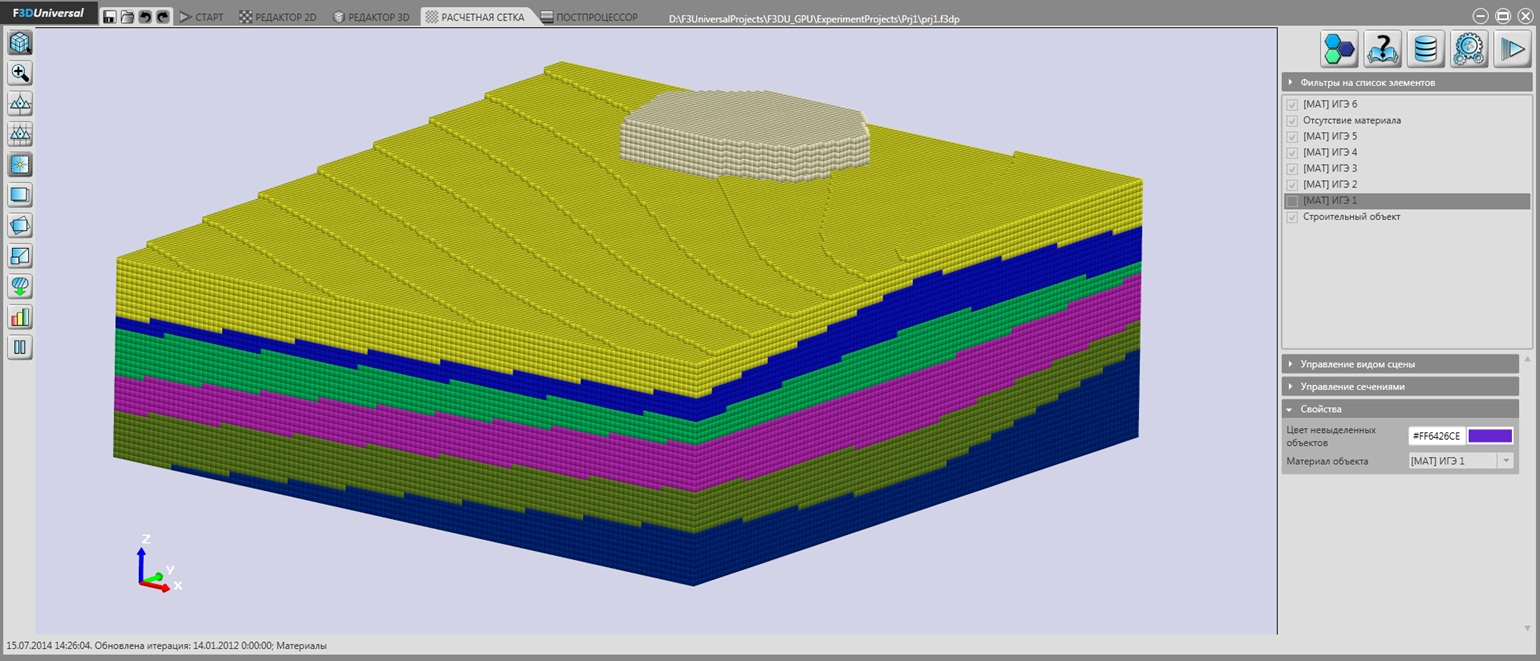Simulation area, discretized by hexahedral mesh

As a result of the numerical computation of the heat equation, a three-dimensional distribution of temperatures is obtained in the simulation area for different moments in time. The thermal field obtained is used for the analysis of thermal regimes of grounds and changes in their mechanical properties. On the basis of these analyses, it is possible to make decisions to ensure the appropriate load bearing capacity of grounds.